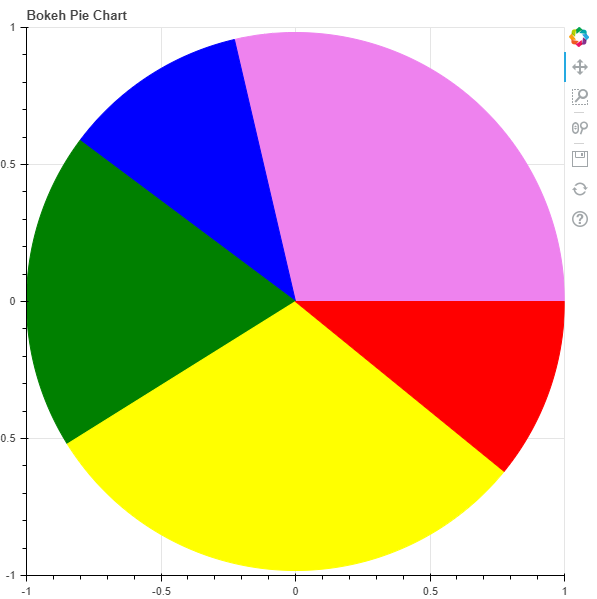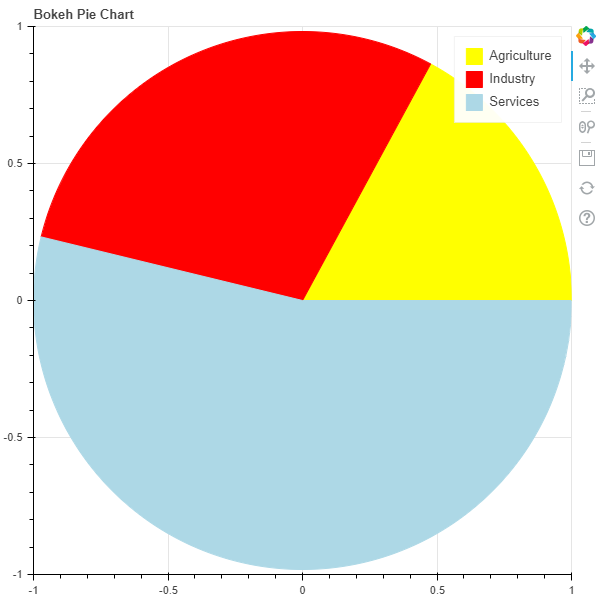Open in App
Not now

# Python Bokeh – Making a Pie Chart

• Last Updated : 28 Jul, 2020

Bokeh is a Python interactive data visualization. It renders its plots using HTML and JavaScript. It targets modern web browsers for presentation providing elegant, concise construction of novel graphics with high-performance interactivity.

Let us see how to plot a pie chart in Bokeh. Does not provide us a module to directly plot a pie chart but we can use the wedge glyphs to make the pie chart. Wedges can be plotted using the `bokeh.plotting.figure.wedge()` function.

In the `wedge()` function, the primary parameters are the `x` and `y `coordinates of the wedge, the `radius`, the `start_angle `and the `end_angle` of the wedge. In order to plot the wedges in such a way that they look like a pie chart, the `x`, `y`, and `radius `parameters of all the wedges will be the same. We will only adjust the `start_angle` and the `end_angle`.

Example 1 :

 `# importing the modules ` `from` `bokeh.plotting ``import` `figure, output_file, show ` ` `  `# file to save the model  ` `output_file(``"gfg.html"``)  ` `          `  `# instantiating the figure object  ` `graph ``=` `figure(title ``=` `"Bokeh Pie Chart"``)  ` ` `  `# center of the pie chart ` `x ``=` `0` `y ``=` `0` ` `  `# radius of the glyphs ` `radius ``=` `1` ` `  `# starting angle values ` `start_angle ``=` `[``0``, ``1.8``, ``2.5``, ` `               ``3.7``, ``5.6``] ` ` `  `# ending angle values ` `end_angle ``=` `[``1.8``, ``2.5``, ``3.7``, ` `             ``5.6``, ``0``] ` ` `  `# color of the wedges ` `color ``=` `[``"violet"``, ``"blue"``, ``"green"``, ` `          ``"yellow"``, ``"red"``] ` ` `  `# plotting the graph ` `graph.wedge(x, y, radius, ` `            ``start_angle, ` `            ``end_angle, ` `            ``color ``=` `color) ` ` `  `# displaying the graph ` `show(graph) `

Output :Example 2 : In his example we will be visualizing some data. In the data we are provided with the Share of sectors in GVA during 2017-18 in India. The shares are :

```Agriculture - 17.1%
Industry - 29.1%
Services - 53.8%
```

To find the `start_angle `and the `end_angle `we will need to convert the percentages into radians using the following formula :

`math.radians((percent / 100) * 360)`

 `# importing the modules ` `from` `bokeh.plotting ``import` `figure, output_file, show ` `import` `math ` ` `  `# file to save the model  ` `output_file(``"gfg.html"``)  ` `          `  `# instantiating the figure object  ` `graph ``=` `figure(title ``=` `"Bokeh Pie Chart"``)  ` ` `  `# name of the sectors ` `sectors ``=` `[``"Agriculture"``, ``"Industry"``, ``"Services"``] ` ` `  `# % tage weightage of the sectors ` `percentages ``=` `[``17.1``, ``29.1``, ``53.8``] ` ` `  `# converting into radians ` `radians ``=` `[math.radians((percent ``/` `100``) ``*` `360``) ``for` `percent ``in` `percentages] ` ` `  `# starting angle values ` `start_angle ``=` `[math.radians(``0``)] ` `prev ``=` `start_angle[``0``] ` `for` `i ``in` `radians[:``-``1``]: ` `    ``start_angle.append(i ``+` `prev) ` `    ``prev ``=` `i ``+` `prev ` ` `  `# ending angle values ` `end_angle ``=` `start_angle[``1``:] ``+` `[math.radians(``0``)] ` ` `  `# center of the pie chart ` `x ``=` `0` `y ``=` `0` ` `  `# radius of the glyphs ` `radius ``=` `1` ` `  `# color of the wedges ` `color ``=` `[``"yellow"``, ``"red"``, ``"lightblue"``] ` ` `  `# plotting the graph ` `for` `i ``in` `range``(``len``(sectors)): ` `    ``graph.wedge(x, y, radius, ` `                ``start_angle ``=` `start_angle[i], ` `                ``end_angle ``=` `end_angle[i], ` `                ``color ``=` `color[i], ` `                ``legend_label ``=` `sectors[i]) ` ` `  `# displaying the graph ` `show(graph) `

Output :My Personal Notes arrow_drop_up
Related Articles